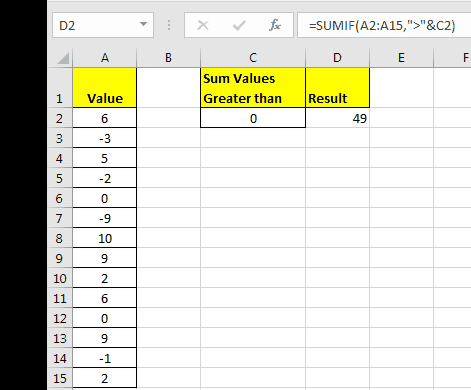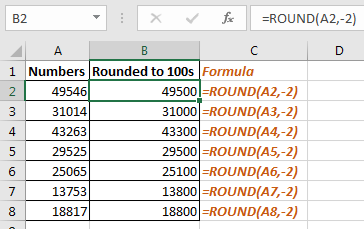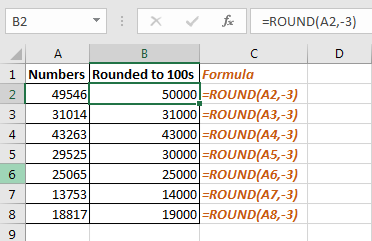# how to Use the Round Function to Round Numbers to Thousands in Excel

While playing with big numbers, all digits of an number doesn’t matter that much and we can ignore them to make it more readable relatable. For example, 1225411 number is more readable when we write it as 1225000. This is called rounding.In this article, we will learn how to round numbers to nearest 100 or 1000.
Generic Formula to Round number to nearest 100

=ROUND(number,-2)

Number: this is the number that you want to round.
-2: this indicates how many number you want to round. Negative sign (-) indicates that you want round from left of the decimal point.

Example 1.

In range A2:A8, I have some numbers. I want to round them to 100. To do so I will write below formula.

=ROUND(A2,-2)

Copy this in below cells.How it works:

Actually when we pass a negative number to ROUND function, it round the numbers before decimal point.

So to round to nearest 1000 we just need to replace -2 to -3 in formula.
Generic Formula to Round number to nearest 100

=ROUND(number,-3)

In same example, if we implement this formula we will get our numbers rounded to nearest 1000.This was quick tutorial of how how to round number to nearest 100 or 1000. Let me know if you have any specific question on this article or any other task related to excel 2016 and VBA. I will be more than happy to help. Till than you may read some related articles.

1.